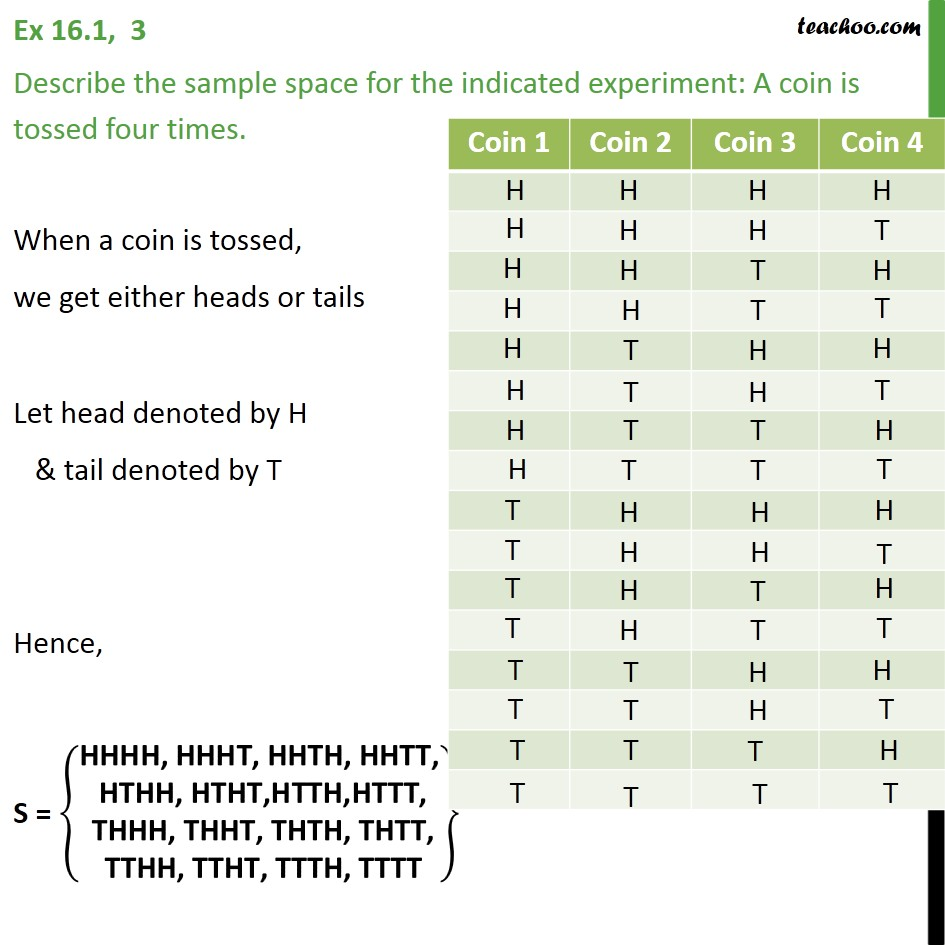1. Chapter 16 Class 11 Probability
2. Concept wise
3. Sample Space

Transcript

Ex 16.1, 3 Describe the sample space for the indicated experiment: A coin is tossed four times. When a coin is tossed, we get either heads or tails Let head denoted by H & tail denoted by T Hence, S = HHHH, HHHT, HHTH, HHTT, HTHH, HTHT,HTTH,HTTT, THHH, THHT, THTH, THTT, TTHH, TTHT, TTTH, TTTT

Sample Space